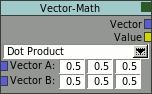#Drag[en]gine Wiki

### Site Tools

epsylon:vegsysnodeedit

For editing the vegetation system to areas can be used in the World Editor. The first one is in the properties area and allows to add, remove and reorder vegetation layers as well as editing their variations. For editing the rule base the node editor is used which can be found in the same area as the 3D view ( click on the Vegetation button on top ). This editor works like most other node based editors and people familiar with those should be immediately familiar with this one. For those new to this kind of editing a small introduction is given.

# Introduction

The node editor bases on representing each rule by a node in a workspace. Each node has a set of input and output slots as well as optionally parameters. The slots are used to connect nodes together. This feeds the output from one node as an input to another node. Depending on which slot such a link connects to different values can be obtained from a node or different parameters set in other nodes. Links can only go from one output slot of one node to one input slot of another node. Yet though multiple links can exist from one node to another as long as at least one of the involved slots are not the same. So an the same input or output node can be connected to multiple other slots. Loops are not allowed and the editor prevents creating links which would produce a loop. Nodes can be of one of three different types: Input, Processing or Output. Input nodes have only output slots and are therefore the start of node chains. Output slot on the other hand only have input slots and are therefore the end of a node chain. There exists only one output rule which is the Result rule. In each layer only one result rule exists. It can not be deleted nor can a second output node be inserted. The last group are the processing nodes which have both input and output slots and are used to process a value on the way through the node chain.

In the vegetation system slots of nodes can transport two kinds of values: vectors or values. A value is a single floating point value while a vector is a 3-component vector containing 3 floating point values. Each slot has exactly one of those two types. They are marked using different colors with yellow for values and a blueish color for vectors. A value type output slot can be connected to a value type or a vector type input slot. If a vector type input slot is the target this is the same as if a vector with all components set to the input value is supplied. This avoids the need for using a combine rule to compose a value into a vector. On the other hand a vector type output slot can only be attached to a vector type input slot. This is due to the fact that a vector can not be mapped to a value in a definitive way. You could use either of the vector components. To avoid this undefined behavior no such connections are allowed and the editor will not allow you to create them in the first place. You can therefore safely connect nodes. If there is an incompatibility the link is drawn in red and will not be created. Valid links are drawn in green and can be created.

Another specialty of this system is the multiplicity of input slots. In most other node systems you can connect only one node to an input slot of a given node. Here you can connect multiple nodes to one input slot of a given node. A few rules can use this to apply an operation on multiple input values. For example the probability input slot on the result rule accepts multiple nodes to be connected. What happens is that all input values are first multiplied and then used as the final input value. This system avoids wild node grids required in other node systems to merge multiple values down into one value. See the explanations on the individual rules below for details on which input slots accept multiple nodes. The editor knows about which input slots understand multiple nodes and will only allow multiple links on those. For slots with only one allowed link the former link will be broken if a new one is created.

Editing the rules is simple to do. Use the right click menu to add nodes to the workspace or to remove the selected one. To move nodes around click on the their title bar to drag them around. To create a link click on an output slot of a node and drag it to an input slot of another node. The link will be created ( if valid ) once you drop on an input slot. Dropping elsewhere stops creating the link. To break one or more links click on the background of the workspace and drag a line across the links you want to cut. To avoid cluttering the workspace each node can be folded. If folded all parameters except the input slots and their names are hidden. To switch between folded and unfolded click on the colored square in the upper right corner of each node. Even using folded nodes the workspace can quickly get too small to hold all nodes. The workspace itself has no bounds so you can place nodes everywhere. To scroll them into view click on the workspace background while holding the Shift key to move it around. You will always see the active vegetation layer in this workspace. To change the layer use the vegetation panel in the properties window to select a different vegetation layer.

# Constant Rule

The constant rule is an Input rule and provides a constant vector to follow up nodes. In addition each component of the constant vector can be tapped directly which turns the constant rule into a cheap 3-value input rule or a 1-value input vector.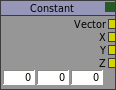# Geometry Rule

The geometry rule is an Input rule and provides informations about the instance the rules are currently evaluated upon. The height output slot contains the absolute y coordinate of the position of the instance in the world and is a value type slot. The normal output slot contains the normal of the ground the instance is sitting upon and is a vector type slot. The terrain type slot returns the type number of the height terrain texture the most dominant or with other words the terrain texture the instance sits upon. This terrain type number can be used to limit plants to grow only on patches of terrain with a given texture. The type number can be chosen freely by the mapper and is not required to be unique for each texture. Usually this number is linked to a math rule with an equal operator. This test works since the equal test is within a small epsilon around the number. Otherwise two math rules with less than and greater than operator can be used. Later solution is useful if the type numbers are laid out in such a way that entire ranges can be checked instead of a single number. The terrain type slot is a value type slot.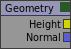# Random Rule

The random rule is an Input rule and provides a random value. The output slot is a value type slot. All nodes tapping from this node receive the same random value. Use different random rule nodes to obtain different random values.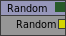# Result Rule

The result rule is the only Output rule and applies the input parameters as the result of the evaluation. The probability input slot takes the probability of the instance coming into existence and is a multiple value input slot. Therefore multiple nodes can be connected in which case all incoming values are multiplied first. This behavior spares a multi-math rule and is the most used one. If no link connects to the probability input slot the value next to it is used instead. The variation input slot takes the number of the variation to apply to the instance if it passes the probability test. The value is in the range of 0 to 1 and maps to the range of 0 to VariationCount as described in the Variation section above. The variation slot accepts only one input link. If the variation input slot has no links the value next to it is used instead. This is the only node existing in a fresh vegetation layer can can not be deleted.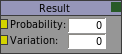# Closest Prop

The closest prop rule is an Input rule and provides informations about the proximity of a certain type of props to the instance the rules are evaluated upon. This rule searches for all objects matching a given object class inside a given search radius and returns informations about the closest one. The distance output slot returns the distance of the closest found prop. If no prop has been found the search distance is returned. This is a value output slot. The direction output slot returns the normalized direction vector to the prop if found. This rule is the basic building stone of natural plant placement providing support for simulating symbiosis or dominance behavior.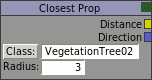# Prop Count

The prop count rule is an Input rule and provides informations about the number of props of a certain type in the vicinity of the instance the rules are evaluated upon. This rule searches for all objects matching a given object class inside a given search radius. The count output slot returns the number of found objects and is a value type slot. Like the closest prop rule the prop count rule is a basic building stone for natural placement and is typically used to locate clusters of objects like for example a group of trees close to each other is a strong indication of a forest.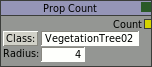# Curve Rule

The curve rule is a Processing rule and transforms an input value using a curve mapping. The input value can host only one link of value type. The incoming value is then mapped using a curve through a series of points. The curve points reside both in X and Y direction inside the range from 0 to 1. Furthermore the curve can be of three interpolation types: constant, linear and cubic. Using the constant interpolation mode the y value of each point extends up to the next point where the value jumps to the y value of the new point. This way a step type mapping can be achieved useful for the variation input slot of the result rule. Using the linear interpolation the curve is a piecewise linear curve. Using the cubic interpolation the curve maps using a cubic interpolation method the values across the points with the curve passing through all of the points. The curve is not required to be monotonically raising or falling. Points can be though not placed closer than 0.001 units apart. The output value is again of value type. To edit the curve click on existing points to move them around. To add a new point click anywhere on the curve while pressing the Shift key. You can directly drag the new point keeping the mouse button pressed. To remove a point click on the point while holding the Control key. The end points are not required to line up with the 0 and 1 boundary. The curve extends to the appropriate boundary according to the interpolation mode selected. ( TODO: add the interpolation combo box… it's still missing but it's in the rule itself already )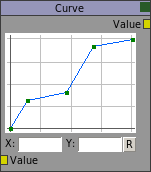# Mapping Rule

The mapping rule is a Processing rule and clamps an input value to a given range. The lower and upper bound are given by the lower and upper input slots which are value type slots accepting only one link. If there are no links on these slots the value next to the slot is used instead. The value input slot provides the value to be mapped and is a value type slot allowing only one link. Values below the lower boundary are clamped to 0. Values above the upper boundary are clamped to 1. All values in between are mapped linearly to the range of 0 to 1. This is sometimes also called compressing the input value. The optional inverted check box allows to flip the output so the mapping is not from 0 to 1 but from 1 to 0 which is something used often. Therefore the lower boundary has always to be lower than the upper boundary. Setting both to the same value or setting lower larger than upper results in a constant 0 from the rule.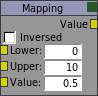# Math Rule

The math rule is a Processing rule and applies a mathematical operation to one or two input values. The operands are given by the value 1 and value 2 input slots which accept only one link of type value. Depending on the selected operation both or only the first value is used. If no links are attached to the slots the value next to the slot is used instead. In the case of an invalid operation like for example dividing by zero a 0 is returned. This rule contains a multitude of operations including arithmetic, trigonometric and boolean operators. For boolean operators the output is 1 if the operation evaluates to true and 0 otherwise.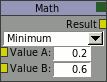# Multi-Math Rule

The multi-math rule is a Processing rule similar to the math rule but takes advantage of the multiplicity of input slots. This rule applies a mathematical operation to a set of input values. Therefore only a small number of operations are supported namely though which are commutative. The input value takes therefore multiple links of value type.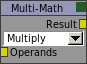# Combine Rule

The combine rule is a Processing rule merging three individual values into a vector. The input slots take a value for each of the vector components and accept only one value type link. If an input value has no links for a given component the value next to the slot is used instead. With no inputs attached this rule behaves similar to the constant rule. This rule is useful to create a vector out of a set of calculated values as there is no other way to obtain a vector form individual values.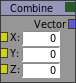# Components Rule

The components rule is a Processing rule decomposing a vector into its components. The input slot takes one vector type link and provides each component as an output value. This rule is useful to obtain a specific component from a vector which is required since linking a vector type output slot to a value type input slot is not allowed.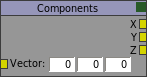# Vector-Math Rule

The vector-math rule is a Processing rule applying a mathematical operation to one or two input vectors. This rule behaves similar to the math rule but operates on vectors. One difference is that some vector operations produce a value instead of a vector. Therefore this rule has two output slots. Depending on which operation is selected one of the two slots returns the result while the other returns a default 0 value or 0 vector. Make sure to pick the right output slot.